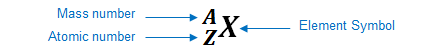# Problem: Which of the following isotopes would you expect to be stable?a. 208Pbb. Carbon-12c. Uranium 235d. 4Hee. 208Po

###### FREE Expert Solution
87% (429 ratings)
###### FREE Expert Solution

We're asked to determine which of the following isotopes are expected to be stable.

Recall that Isotopes represent atoms of an element that contain the same atomic number (same number of protons), but different mass numbers (different number of neutrons) and can be written asIn terms of stability of isotopes, we use the neutrons to protons (n/p) ratio:

• Isotopes with neutrons to protons (n/p) ratio close to 1 are considered stable
• For atoms with lower atomic numbers
• n = p  (n/p)  ≈ 1
• Isotopes with neutrons to protons (n/p) ratio greater than 1 are considered unstable
• For atoms with higher atomic numbers
• n > p (n/p) > 1

For this problem, we need to do the following steps:

Step 1: Determine the # of protons, p based on the atomic number of each isotope using the Periodic Table

Recall that the atomic # = # of protons (p)

Step 2: Determine the # of neutrons, n for each isotope

Recall that the mass # = # of neutrons (n) + # of protons (p)

Step 3: Calculate the n/p ratio to determine its stability

87% (429 ratings)###### Problem Details

Which of the following isotopes would you expect to be stable?

a. 208Pb

b. Carbon-12

c. Uranium 235

d. 4He

e. 208Po